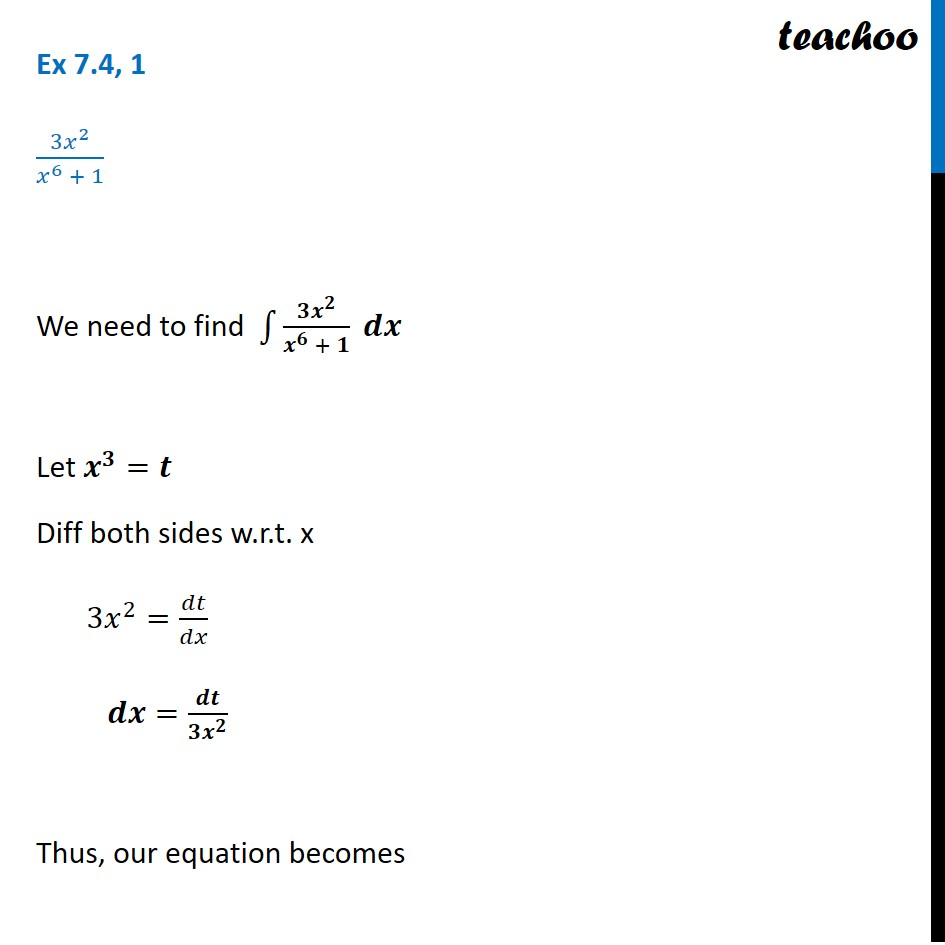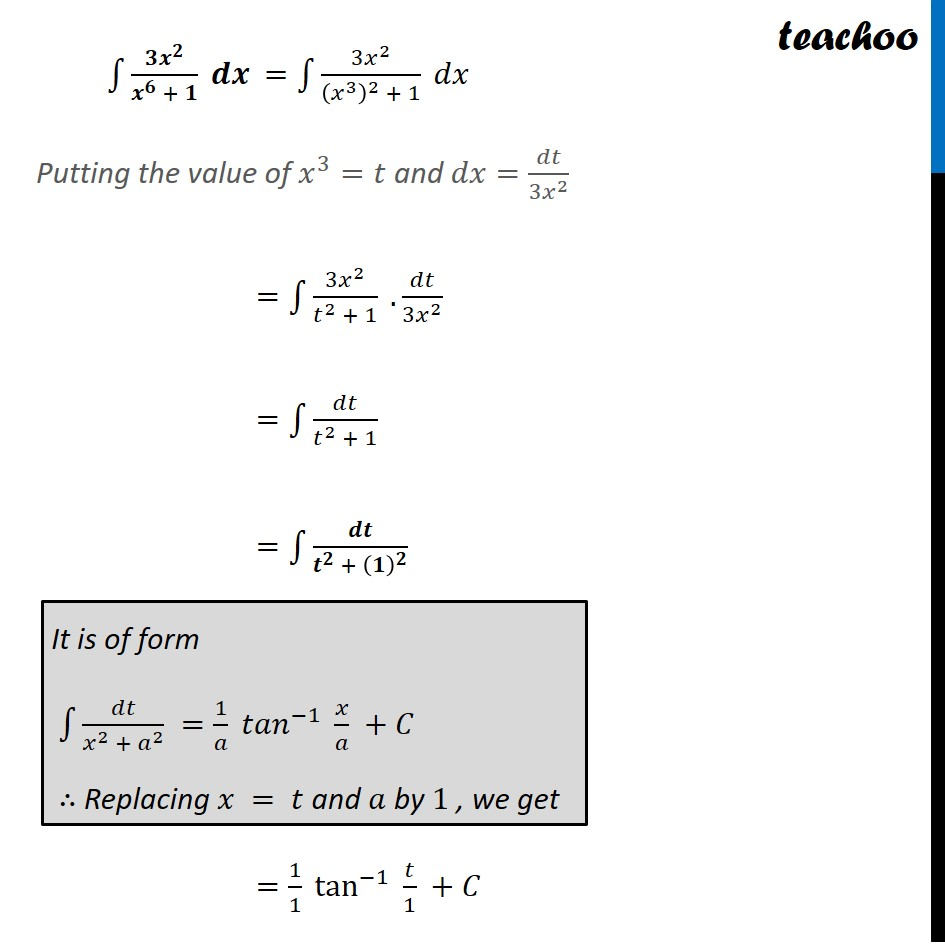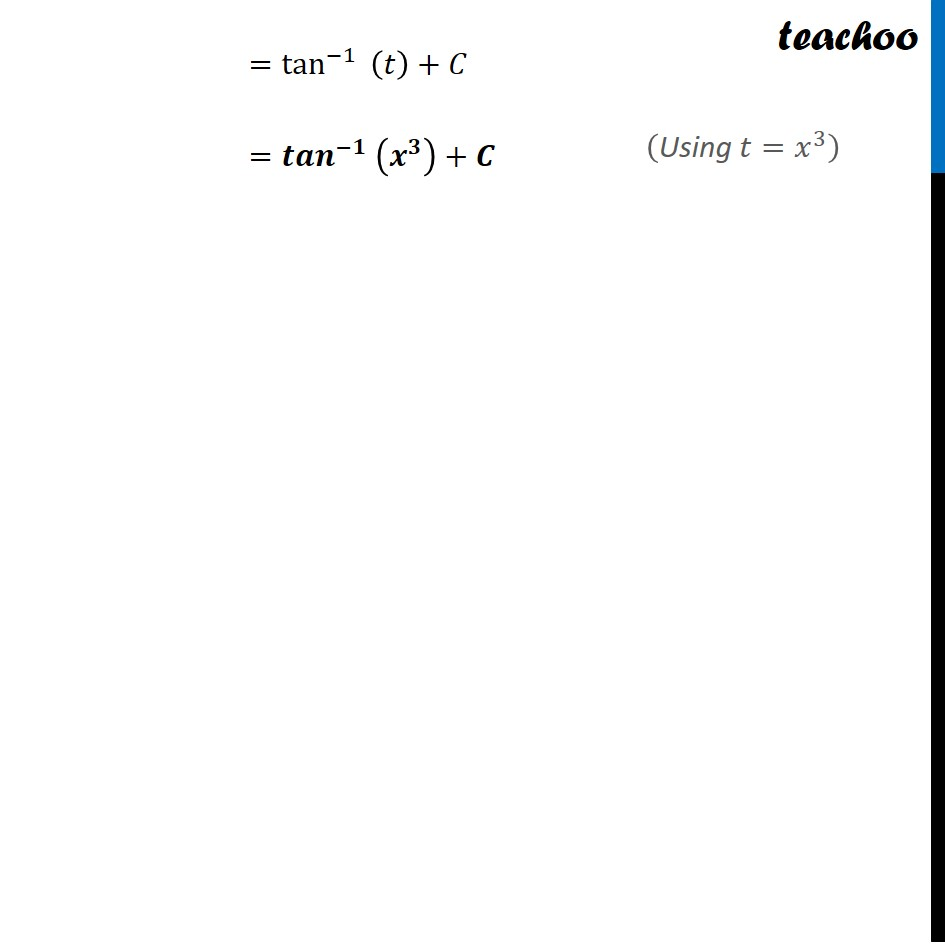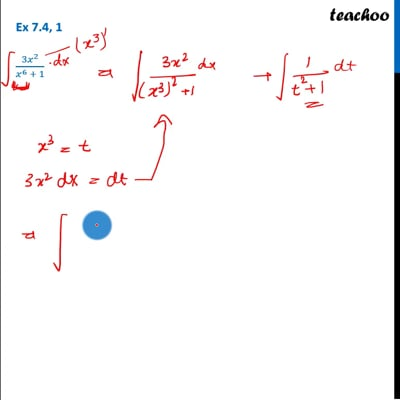Integration by specific formulaes - Formula 3

Chapter 7 Class 12 Integrals
Concept wiseThis video is only available for Teachoo black users

Learn in your speed, with individual attention - Teachoo Maths 1-on-1 Class

### Transcript

Ex 7.4, 1 (3𝑥^2)/(𝑥^6 + 1) We need to find ∫1▒(𝟑𝒙^𝟐)/(𝒙^𝟔 + 𝟏) 𝒅𝒙 Let 𝒙^𝟑=𝒕 Diff both sides w.r.t. x 3𝑥^2=𝑑𝑡/𝑑𝑥 𝒅𝒙=𝒅𝒕/(𝟑𝒙^𝟐 ) Thus, our equation becomes ∫1▒(𝟑𝒙^𝟐)/(𝒙^𝟔 + 𝟏) 𝒅𝒙 =∫1▒(3𝑥^2)/((𝑥^3 )^2 + 1) 𝑑𝑥 Putting the value of 𝑥^3=𝑡 and 𝑑𝑥=𝑑𝑡/(3𝑥^2 ) =∫1▒(3𝑥^2)/(𝑡^2 + 1) .𝑑𝑡/(3𝑥^2 ) =∫1▒𝑑𝑡/(𝑡^2 + 1) =∫1▒𝒅𝒕/(𝒕^𝟐 + (𝟏)^𝟐 ) =1/1 tan^(−1)⁡〖 𝑡/1 〗+𝐶 It is of form ∫1▒𝑑𝑡/(𝑥^2 + 𝑎^2 ) =1/𝑎 〖〖𝑡𝑎𝑛〗^(−1) 〗⁡〖𝑥/𝑎〗 +𝐶 ∴ Replacing 𝑥 = 𝑡 and 𝑎 by 1 , we get =tan^(−1)⁡〖 (𝑡)〗+𝐶 =〖〖𝒕𝒂𝒏〗^(−𝟏) 〗⁡(𝒙^𝟑 )+𝑪 ("Using" 𝑡=𝑥^3 )